Cody

# Problem 42699. Find the Area of a Polygon

Solution 2061084

Submitted on 17 Dec 2019 by Asif Newaz
This solution is locked. To view this solution, you need to provide a solution of the same size or smaller.

### Test Suite

Test Status Code Input and Output
1   Pass
A = [0 0; 120 120; 120 0]; y_correct = 7200; assert(isequal(area_of_polygon(A),y_correct))

b = polyshape with properties: Vertices: [3×2 double] NumRegions: 1 NumHoles: 0 y = 7200

`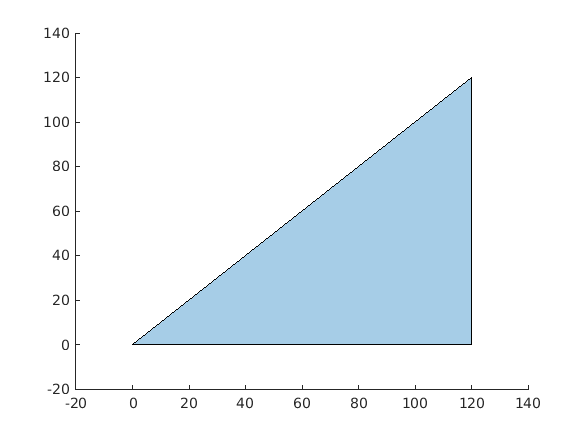`
2   Pass
A = [1 2; 12 3; 6 7]; y_correct = 25; assert(isequal(area_of_polygon(A),y_correct))

b = polyshape with properties: Vertices: [3×2 double] NumRegions: 1 NumHoles: 0 y = 25

`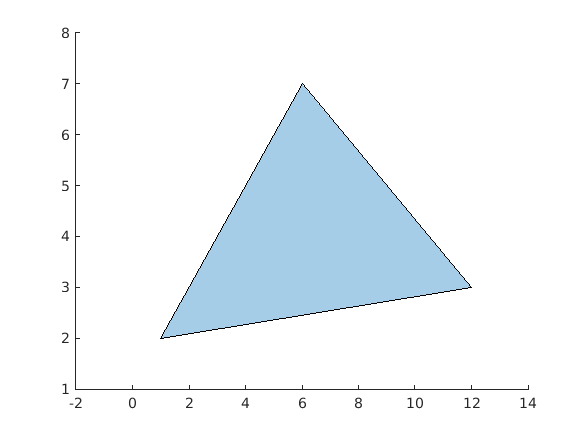`
3   Pass
A = [0 0; 0 100; 100 100; 100 0]; y_correct = 10000; assert(isequal(area_of_polygon(A),y_correct))

b = polyshape with properties: Vertices: [4×2 double] NumRegions: 1 NumHoles: 0 y = 10000

`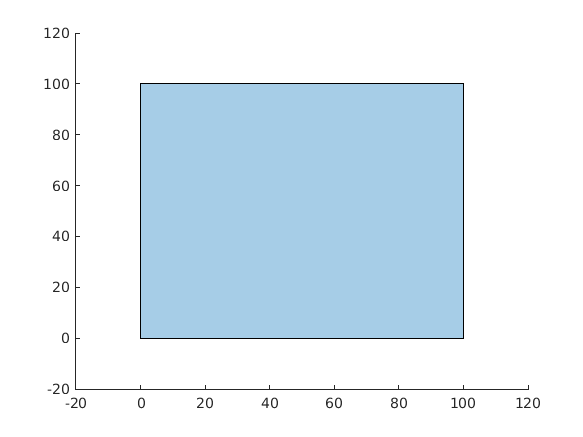`
4   Pass
A = [0 0; 0 200; 200 200; 200 0]; y_correct = 40000; assert(isequal(area_of_polygon(A),y_correct))

b = polyshape with properties: Vertices: [4×2 double] NumRegions: 1 NumHoles: 0 y = 40000

`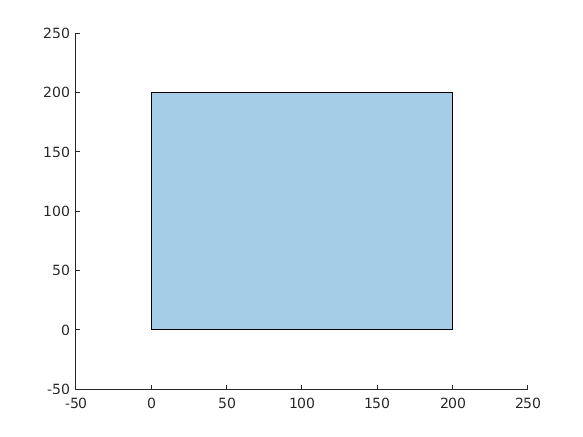`
5   Pass
A = [0 0; 0 50; 100 100; 100 0]; y_correct = 7500; assert(isequal(area_of_polygon(A),y_correct))

b = polyshape with properties: Vertices: [4×2 double] NumRegions: 1 NumHoles: 0 y = 7500

`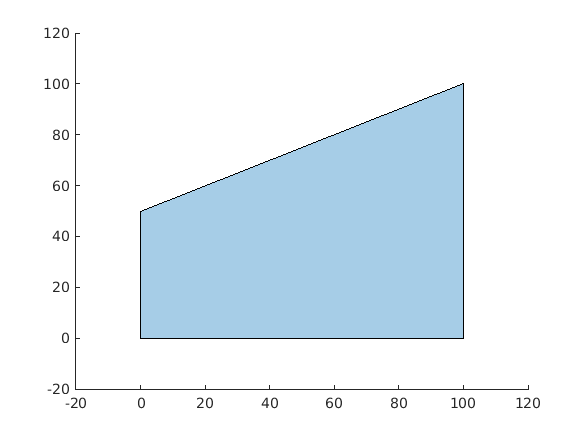`
6   Pass
A = [0 0; 200 100; 500 500; 400 1000; 100 300; -100 100; -300 -200]; y_correct = 230000; assert(isequal(area_of_polygon(A),y_correct))

b = polyshape with properties: Vertices: [7×2 double] NumRegions: 1 NumHoles: 0 y = 230000

`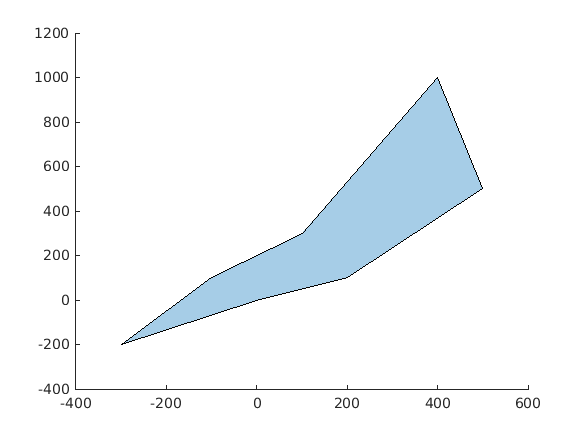`

### Community Treasure Hunt

Find the treasures in MATLAB Central and discover how the community can help you!

Start Hunting!